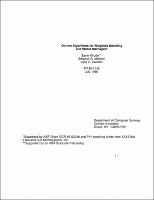## On-line Algorithms for Weighted Matching and Stable Marriages##### Abstract
We give an on-line deterministic algorithm for the bipartite weighted matching problem that achieves a competitive ratio of $O(n)$. In fact, this algorithm is almost optimal - the lower bound on the performance ratio of any deterministic online algorithm is $\Omega (n/ \sqrt{log n})$. We also study the stable marriage problem, where we are interested in the number of unstable pairs produced. We show that the simple "first come, first served" deterministic algorithm yields on the average $O(n$ log $n$) unstable pairs, but in the worst case no deterministic or randomized on-line algorithm can do better that $\Omega(n^{2})$ unstable pairs.
1990-07
##### Publisher
Cornell University
##### Keywords
computer science; technical report
##### Previously Published As
http://techreports.library.cornell.edu:8081/Dienst/UI/1.0/Display/cul.cs/TR90-1143
technical report